Ch 5. Rigid Body General Motion Multimedia Engineering Dynamics Fixed Axis Rotation Plane Motion Velocities Zero Velocity Point Plane Motion Accelerations Multiple Gears Rot. Coord. Velocities Rot. Coord. Acceleration
 Chapter - Particle - 1. General Motion 2. Force & Accel. 3. Energy 4. Momentum - Rigid Body - 5. General Motion 6. Force & Accel. 7. Energy 8. Momentum 9. 3-D Motion 10. Vibrations Appendix Basic Math Units Basic Equations Sections Search eBooks Dynamics Fluids Math Mechanics Statics Thermodynamics Author(s): Kurt Gramoll ©Kurt GramollDYNAMICS - THEORY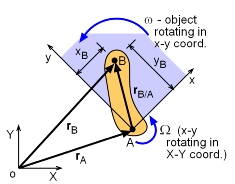Position Vectors

The acceleration of a moving point on a rotating rigid body is more complex than its velocity since there are tangent and normal terms.

To determine the acceleration of a rotating rigid body with a moving point start with the relative velocity vector equation that was derived in the previous Rotating Coordinates:Velocities section. To help with modeling complex systems, two coordinate systems are used: X-Y is fixed and x-y rotates in the X-Y system. Note, x-y system is not fixed to the body.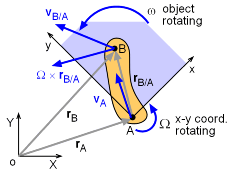Velocity Vectors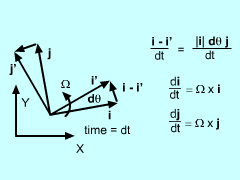Time Derivative of i and j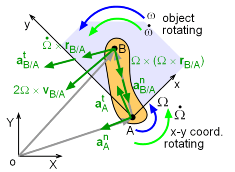Acceleration Vectors

Start with the relative velocity equation for a moving frame of reference:

vB = vA + Ω × rB/A + (vB/A)rel

Differentiate with respect to time:

aB = aA + dΩ/dt × rB/A + Ω × drB/A/dt + dvB/A/dt

Similar to velocities, the x-y coordinate system is rotating inside the X-Y coordinate system. This means the time derivatives of the unit vectors, di/dt and dj/dt are not zero but

di/dt = Ω × i
dj/dt = Ω × j

Thus, the time derivative of the position vector, rB/A is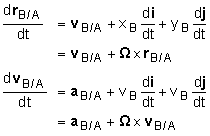Putting all the terms together gives,

 aB =aA + dΩ/dt × rB/A + Ω × (Ω × rB/A)  + 2 Ω × (vB/A)rel + (aB/A)rel

Coordinate Transformation

Since two coordinates are involved with most rotating coordinate system problems, the two systems need to be related. In other words, one coordinate system needs to be mapped into the other, and vice versa. Relating two coordinate system is a common task in most all engineering fields, not just dynamics.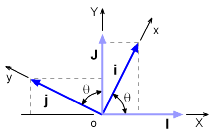Velocity vectors

The easiest way to relate coordinate systems is to describe one system in the other, just like is done for a point or vector. For example, the i unit-vector i can be described in the coordinate system XY as

i = cosθ I + sinθ J

likewise, the j unit-vector is

j = -sinθ I + cosθ

These relationships can be inverted to describe I and J using i and j unit-vectors

I =  cosθ I - sinθ J
J = sinθ I  + cosθ J

These transformation equations are commonly written in matrix form, and called the 2D coordinate transformation matrix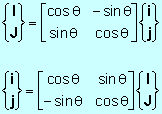They can be used for any two coordinate systems where the xy-system is rotated an angle θ relative to the XY-system.

Practice Homework and Test problems now available in the 'Eng Dynamics' mobile app
Includes over 400 problems with complete detailed solutions.
Available now at the Google Play Store and Apple App Store.